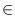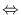Next: Shortest Path Algorithms ( Up: Graph Algorithms Previous: Graph Algorithms   Contents   Index

# Basic Graph Algorithms ( basic_graph_alg )

 bool TOPSORT(const graph& G, node_array& ord) TOPSORT takes as argument a directed graph G(V, E). It sorts G topologically (if G is acyclic) by computing for every node vV an integer ord[v] such that 1 < = ord[v] < = | V| and ord[v] < ord[w] for all edges (v, w)E. TOPSORT returns true if G is acyclic and false otherwise. The algorithm () has running time O(| V| + | E|). bool TOPSORT(const graph& G, list& L) a variant of TOPSORT that computes a list L of nodes in topological order (if G is acyclic). It returns true if G is acyclic and false otherwise. bool TOPSORT1(graph& G) a variant of TOPSORT that rearranges nodes and edges of G in topological order (edges are sorted by the topological number of their target nodes). list DFS(const graph& G, node s, node_array& reached) DFS takes as argument a directed graph G(V, E), a node s of G and a node_array reached of boolean values. It performs a depth first search starting at s visiting all reachable nodes v with reached[v] = false. For every visited node v reached[v] is changed to true. DFS returns the list of all reached nodes. The algorithm () has running time O(| V| + | E|). list DFS_NUM(const graph& G, node_array& dfsnum, node_array& compnum) DFS_NUM takes as argument a directed graph G(V, E). It performs a depth first search of G numbering the nodes of G in two different ways. dfsnum is a numbering with respect to the calling time and compnum a numbering with respect to the completion time of the recursive calls. DFS_NUM returns a depth first search forest of G (list of tree edges). The algorithm () has running time O(| V| + | E|). list BFS(const graph& G, node s, node_array& dist) BFS takes as argument a directed graph G(V, E),a node s of G and a node array dist of integers. It performs a breadth first search starting at s visiting all nodes v with dist[v] = - 1 reachable from s. The dist value of every visited node is replaced by its distance to s. BFS returns the list of all visited nodes. The algorithm () has running time O(| V| + | E|). list BFS(const graph& G, node s, node_array& dist, node_array& pred) performs a bread first search as described above and computes for every node v the predecessor edge pred[v] in the bfs shortest path tree. (You can use the function COMPUTE_SHORTEST_PATH to extract paths from the tree (cf. Section shortest_path).) int COMPONENTS(const graph& G, node_array& compnum) COMPONENTS takes a graph G(V, E) as argument and computes the connected components of the underlying undirected graph, i.e., for every node vV an integer compnum[v] from [0...c - 1] where c is the number of connected components of G and v belongs to the i-th connected component iff compnum[v] = i. COMPONENTS returns c. The algorithm () has running time O(| V| + | E|). int STRONG_COMPONENTS(const graph& G, node_array& compnum) STRONG_COMPONENTS takes a directed graph G(V, E) as argument and computes for every node vV an integer compnum[v] from [0...c - 1] where c is the number of strongly connected components of G and v belongs to the i-th strongly connected component iff compnum[v] = i. STRONG_COMPONENTS returns c. The algorithm () has running time O(| V| + | E|). int BICONNECTED_COMPONENTS(const graph& G, edge_array& compnum) BICONNECTED_COMPONENTS computes the biconnected components of the undirected version of G. A biconnected component of an undirected graph is a maximal biconnected subgraph and a biconnected graph is a graph which cannot be disconnected by removing one of its nodes. A graph having only one node is biconnected. Let c be the number of biconnected component and let c' be the number of biconnected components containing at least one edge, c - c' is the number of isolated nodes in G, where a node v is isolated if is not connected to a node different from v (it may be incident to self-loops). The function returns c and labels each edge of G (which is not a self-loop) by an integer in [0...c' - 1]. Two edges receive the same label iff they belong to the same biconnected component. The edge labels are returned in compnum. Be aware that self-loops receive no label since self-loops are ignored when interpreting a graph as an undirected graph. The algorithm () has running time O(| V| + | E|). GRAPH TRANSITIVE_CLOSURE(const graph& G) TRANSITIVE_CLOSURE takes a directed graph G = (V, E) as argument and computes the transitive closure of G. It returns a directed graph G' = (V', E') such that G'.inf(.) is a bijective mapping from V' to V and (v, w)E'there is a path from G'.inf(v') to G'.inf(w') in G. (The edge information of G' is undefined.) The algorithm () has running time O(| V|*| E|). GRAPH TRANSITIVE_REDUCTION(const graph& G) TRANSITIVE_REDUCTION takes a directed graph G = (V, E) as argument and computes the transitive reduction of G. It returns a directed graph G' = (V', E'). The function G'.inf(.) is a bijective mapping from V' to V. The graph G and G' have the same reachability relation, i.e. there is a path from v' to w' in G'there is a path from G'.inf(v') to G'.inf(w') in G. And there is no graph with the previous property and less edges than G'. (The edge information of G' is undefined.) The algorithm () has running time O(| V|*| E|). void MAKE_TRANSITIVELY_CLOSED(graph& G) MAKE_TRANSITIVELY_CLOSED transforms G into its transitive closure by adding edges. void MAKE_TRANSITIVELY_REDUCED(graph& G) MAKE_TRANSITIVELY_REDUCED transforms G into its transitive reduction by removing edges.Next: Shortest Path Algorithms ( Up: Graph Algorithms Previous: Graph Algorithms   Contents   Index